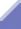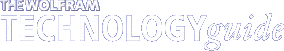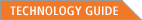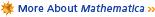Numerical-Precision Tracking (NPT)When repeatedly evaluating x = 11 x - 7, precision is lost at each step. This iteration is evaluated from a starting value of 0.7 (with 16-digit precision) in both Mathematica and a non-Mathematica system, and should result in 0.7 at each step. Mathematica tracks the precision loss, outputting only justifiable digits; the non-Mathematica system does not, leading to a highly misleading result.

Any numerical package or programming language can give numerical results up to machine precision, but only Mathematica will also automatically track and communicate how many digits of the result are accurate--using numerical-precision tracking (NPT) technology. This gives almost complete protection from numerical errors, be they round-off errors or from badly conditioned systems.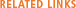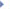Technology Guide: Extended-precision computation, numerical-precision control--specified input mode, numerical-precision control--specified output modeFunction documentation: Accuracy, N, PrecisionThe Mathematica Book: Sections 1.1.2, 1.1.4, 3.1.4